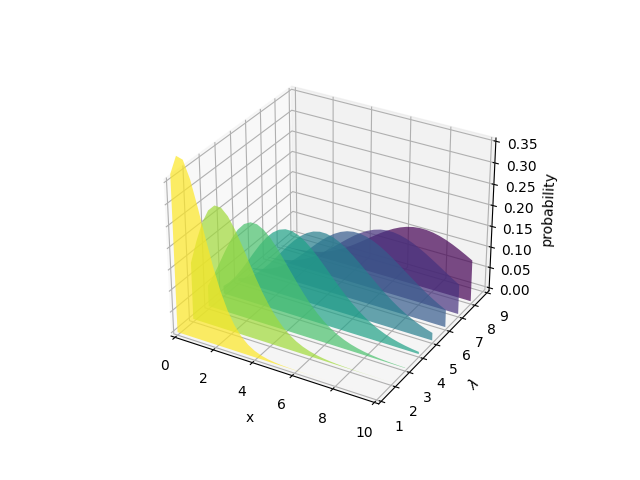# Generate polygons to fill under 3D line graph#

Demonstrate how to create polygons which fill the space under a line graph. In this example polygons are semi-transparent, creating a sort of 'jagged stained glass' effect.from matplotlib.collections import PolyCollection
import matplotlib.pyplot as plt
import math
import numpy as np

# Fixing random state for reproducibility
np.random.seed(19680801)

def polygon_under_graph(x, y):
"""
Construct the vertex list which defines the polygon filling the space under
the (x, y) line graph. This assumes x is in ascending order.
"""
return [(x, 0.), *zip(x, y), (x[-1], 0.)]

x = np.linspace(0., 10., 31)
lambdas = range(1, 9)

# verts[i] is a list of (x, y) pairs defining polygon i.
gamma = np.vectorize(math.gamma)
verts = [polygon_under_graph(x, l**x * np.exp(-l) / gamma(x + 1))
for l in lambdas]
facecolors = plt.colormaps['viridis_r'](np.linspace(0, 1, len(verts)))

poly = PolyCollection(verts, facecolors=facecolors, alpha=.7)
xlabel='x', ylabel=r'$\lambda$', zlabel='probability')